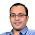## May 3, 2013

### Equations Puzzle

Source: Interview Street

Problem:
Find the number of positive integral solutions for the equations

(1/x) + (1/y) = 1/N!

(read 1 by x plus 1 by y is equal to 1 by n factorial)

Print a single integer which is the no of positive integral solutions modulo 1000007.

Update (24 June 2014):
Solution: Posted by JDGM (James D G Miles) in comments!

1.N, x, y > 0 so we may multiply both sides by N!xy:

N!y + N!x = xy

0 = xy - N!x - N!y = (x-N!)(y-N!) - N!N!

(x-N!)(y-N!) = N!N!

Both factors on the left are positive as x, y > 0 and hence x, y > N! otherwise the product would be non-positive or too small.

The problem is now how many distinct ways the factors of N!N! may be shared between (x-N!) and (y-N!). We may consider just the possible factors in (x-N!) because whatever is left over will be in (y-N!).

Decompose N! into prime powers p₁ᵅ¹p₂ᵅ²...pᵢᵅⁱ then we may have between zero and the full 2α₁ of N!N!'s p₁ powers in the (x-N!) factor and the rest in the (y-N!) factor.

And so on, for p₂, p₃, ..., pᵢ.

The number of solutions is therefore (2α₁+1)(2α₂+1)...(2αᵢ+1) because for each power p we have 2α+1 choices available to us as to how it shall be split between (x-N!) and (y-N!).

It is straightforward to write an algorithm to find the α values of N!'s prime factorisation for given N, and hence calculate the answer using the preceding formula.

For the second part, are we to similarly take arbitrary N, or is the solution to be taken across all values of x, y, N in Z₁₀₀₀₀₀₇? There can only be at most a finite 1000007³ such combinations of x, y, N so the solution in the latter case would be defined.

Anyway, choose arbitrary M = 1/N! mod 1000007 and we require the number of solutions to 1/x + 1/y = M mod 1000007.

My algorithm here is to tally ab=1 mod 1000007 for 0<a≤b<1000007 then build an array of that size and fill it with the a values for which ab=1 mod 1000007 through all compliant b. These are the possible 1/x and 1/y values - crucially note that 1/x or 1/y is not necessarily defined for all x, y in Z₁₀₀₀₀₀₇ which is why this step is necessary. The algorithm has effectively calculated just over half of the Z₁₀₀₀₀₀₇ multiplication table (includes diagonal) and stored the inverses where they exist, by picking out the corresponding factor where the entry in a row is 1. The next step of my algorithm is to go through this array, looking for values also in the array which add to the current value to make M mod 1000007. For each it finds, it adds 2 to the total number of solutions (because there is also a solution in the table on the other side of the diagonal - the table is symmetric), except in the case where the value lies on the diagonal when it adds only 1 to the total number of solutions (1000007 is odd, so the diagonal is its own reflection).

If the question requires the number of all solutions over all x, y, N then we may repeat for all 0 < 1/N! < 1000007 and sum them. If not, and there is a single solution for all N then one calculation will suffice, though one may wish to calculate them all anyway to prove that they are the same.

I believe Interview Street does not wish its answers publicly published so let's leave it there.

1.Thanks a ton. Nice solution

2.I do not know ... it is infinite? Because if
x = 2 * N! and
y = 2 * N!

Then for any N greater than 2 it will be an equality.
As many N, those many solutions.
So infinite

1.That's fair Vivek, but I believe the convention with this type of question is that capital-letter N is an arbitrary input for an algorithm, and not a variable of the problem in the same sense as x and y.

2.This comment has been removed by the author.

3.And so, answer will be a function of N.

3.Solving for a fixed N.

1/a = 1/(a+k) + k/a(a+k)...........(1)

If a(a+k)/k is an integer, we have a solution for 1/a = 1/a+k + 1/b, where b = a(a+k)/k

Therefore in this case, we are looking for all k such that N!(N!+k)/k is an integer.

Basically this means k divides N!^2

So we need the number of divisors of N!^2.

One way of calculating the power of p in the decompsoition of N! is the famous expression
a(p) = sum over j((n/p) + n/p^2)....+ (n/p^j)+....)
Based on this, we know a(p) for all the primes, and therefore the number of divisors is
(product over all primes(2a(p)+1) - 1)/2
(Divide by 2 to account for double counting)

1.You do not need to divide by 2. There is no double counting here as (1,2) is different from (2,1) when (x,y) is the solution to an equation. Thanks

2.Also, -1 is also not needed, as the trivial solution is also acceptable here. Thanks

### Fraction Brainteaser

Source: Sent to me by Gaurav Sinha Problem: Siddhant writes a Maths test and correctly answers 5 out of 6 Arithmetic questions and 20...